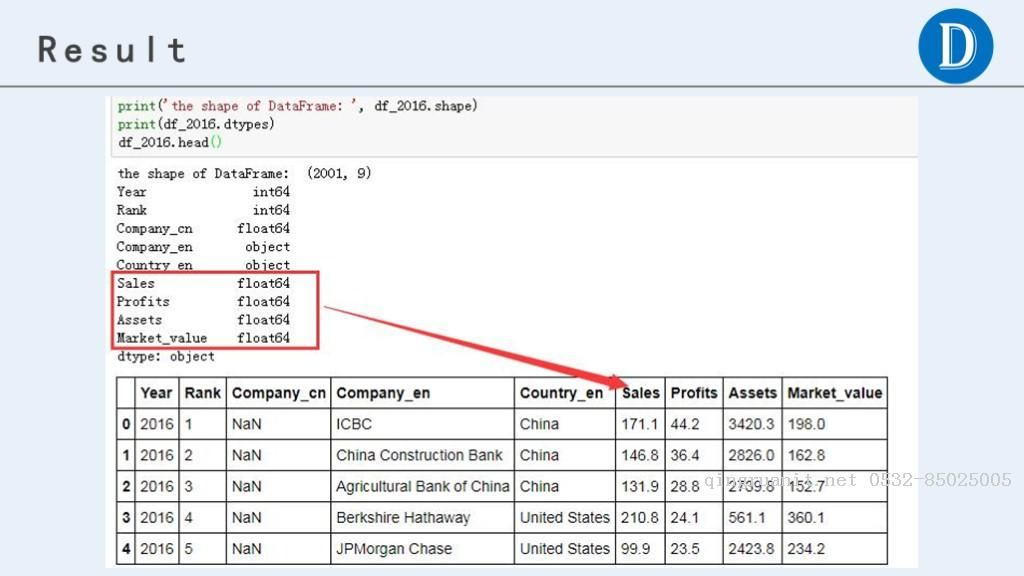• windows 7， 64位

• python 3.5

• pandas 0.19.2版本# 1 处理方法 Method-1

• 加载数据，并添加列的名称

```import pandas as pd

df_2016 = pd.read_csv('data_2016.csv', encoding='gbk',header=None)# 更新列名df_2016.columns = ['Year', 'Rank', 'Company_cn','Company_en',                   'Country_en', 'Sales', 'Profits', 'Assets', 'Market_value']

print('the shape of DataFrame: ', df_2016.shape)
print(df_2016.dtypes)
• 获取单位为十亿（’B’）的数据

```# 数据单位为 B的数据（Billion，十亿）df_2016_b = df_2016[df_2016['Sales'].str.endswith('B')]
print(df_2016_b.shape)
df_2016_b```
• 获取单位为百万(‘M’)的数据

```# 数据单位为 M的数据（Million，百万）df_2016_m = df_2016[df_2016['Sales'].str.endswith('M')]
print(df_2016_m.shape)
df_2016_m```

# 2 处理方法 Method-2

## 2.3 处理’M’相关的数据

（2）替换字符串“M”为空值

（3）用pd.to_numeric()转换为数字

（4）除以1000，转换为十亿美元，与其他行的数据一致

```# 替换美元符号df_2016['Sales'] = df_2016['Sales'].str.replace('\$','')# # 查看异常值，均为字母（“undefined”）# df_2016[df_2016['Sales'].str.isalpha()]# 替换异常值“undefined”为空白# df_2016['Sales'] = df_2016['Sales'].str.replace('undefined','')df_2016['Sales'] = df_2016['Sales'].str.replace('^[A-Za-z]+\$','')# 替换符号十亿美元“B”为空白，数字本身代表的就是十亿美元为单位df_2016['Sales'] = df_2016['Sales'].str.replace('B','')# 处理含有百万“M”为单位的数据，即以“M”结尾的数据# 思路：# （1）设定查找条件mask；# （2）替换字符串“M”为空值# （3）用pd.to_numeric()转换为数字# （4）除以1000，转换为十亿美元，与其他行的数据一致mask = df_2016['Sales'].str.endswith('M')
print('the shape of DataFrame: ', df_2016.shape)
print(df_2016.dtypes)

# 3 处理方法 Method-3

## 3.2 编写数据处理的自定义函数

```def pro_col(df, col):
# 替换相关字符串，如有更多的替换情形，可以自行添加
df[col] = df[col].str.replace('\$','')
df[col] = df[col].str.replace('^[A-Za-z]+\$','')
df[col] = df[col].str.replace('B','')    # 注意这里是'-\$'，即以'-'结尾，而不是'-'，因为有负数
df[col] = df[col].str.replace('-\$','')
df[col] = df[col].str.replace(',','')    # 处理含有百万“M”为单位的数据，即以“M”结尾的数据
# 思路：
# （2）替换字符串“M”为空值
# （3）用pd.to_numeric()转换为数字
# （4）除以1000，转换为十亿美元，与其他行的数据一致

# 将字符型的数字转换为数字类型
df[col] = pd.to_numeric(df[col])    return df```

## 3.3 将自定义函数进行应用

```pro_col(df_2016, 'Sales')
pro_col(df_2016, 'Profits')
pro_col(df_2016, 'Assets')
pro_col(df_2016, 'Market_value')

print('the shape of DataFrame: ', df_2016.shape)
print(df_2016.dtypes)

```cols = ['Sales', 'Profits', 'Assets', 'Market_value']for col in cols:
pro_col(df_2016, col)

print('the shape of DataFrame: ', df_2016.shape)
print(df_2016.dtypes)